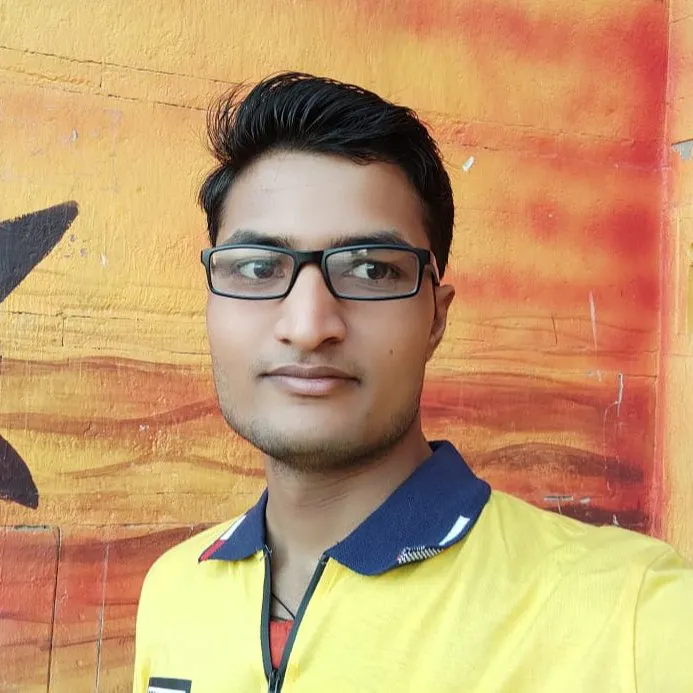# Arduino DS1307 RTC CLOCK

Arduino RTC digital CLOCK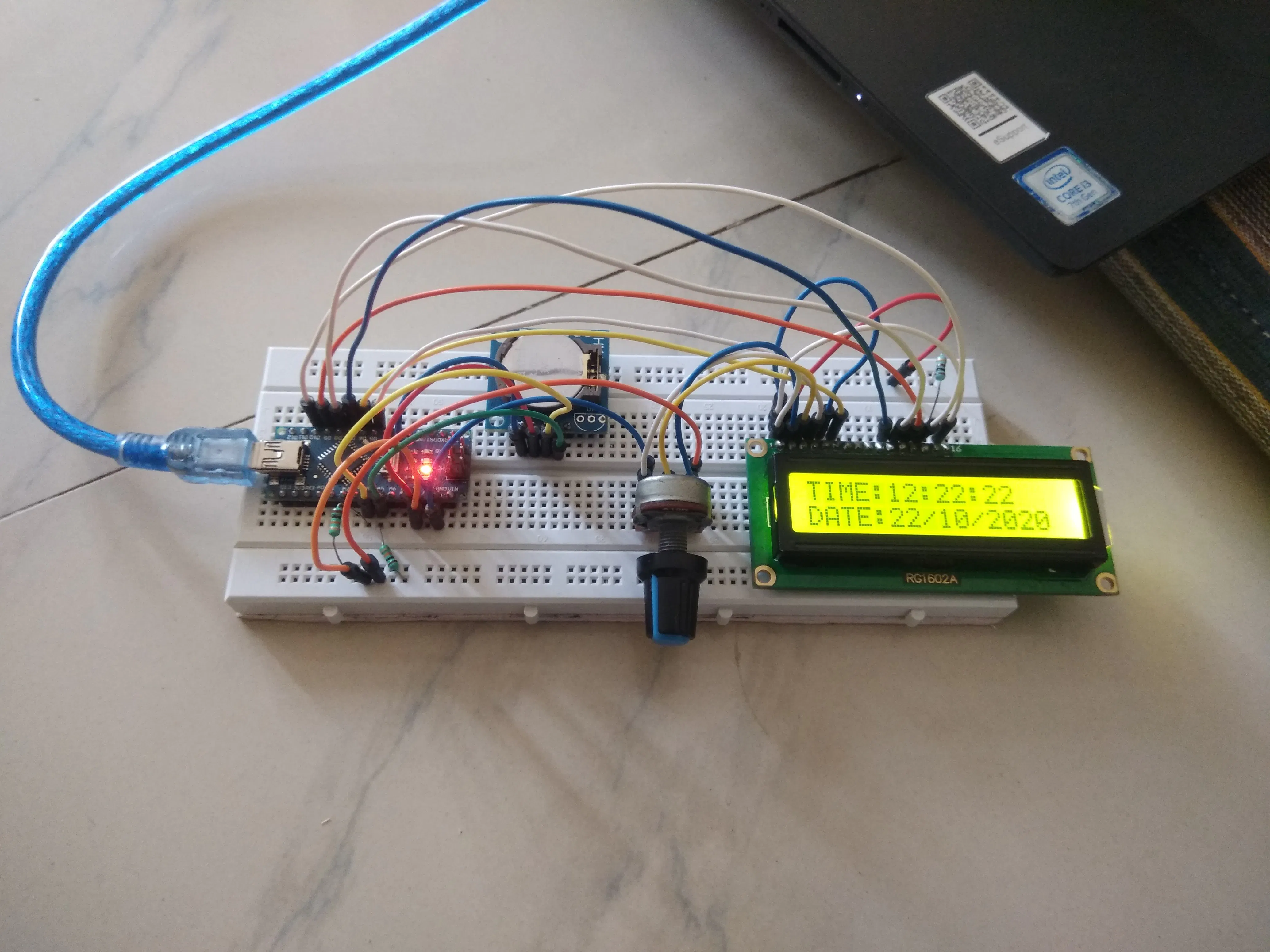## Things used in this project

### Hardware componentsArduino Nano R3
×1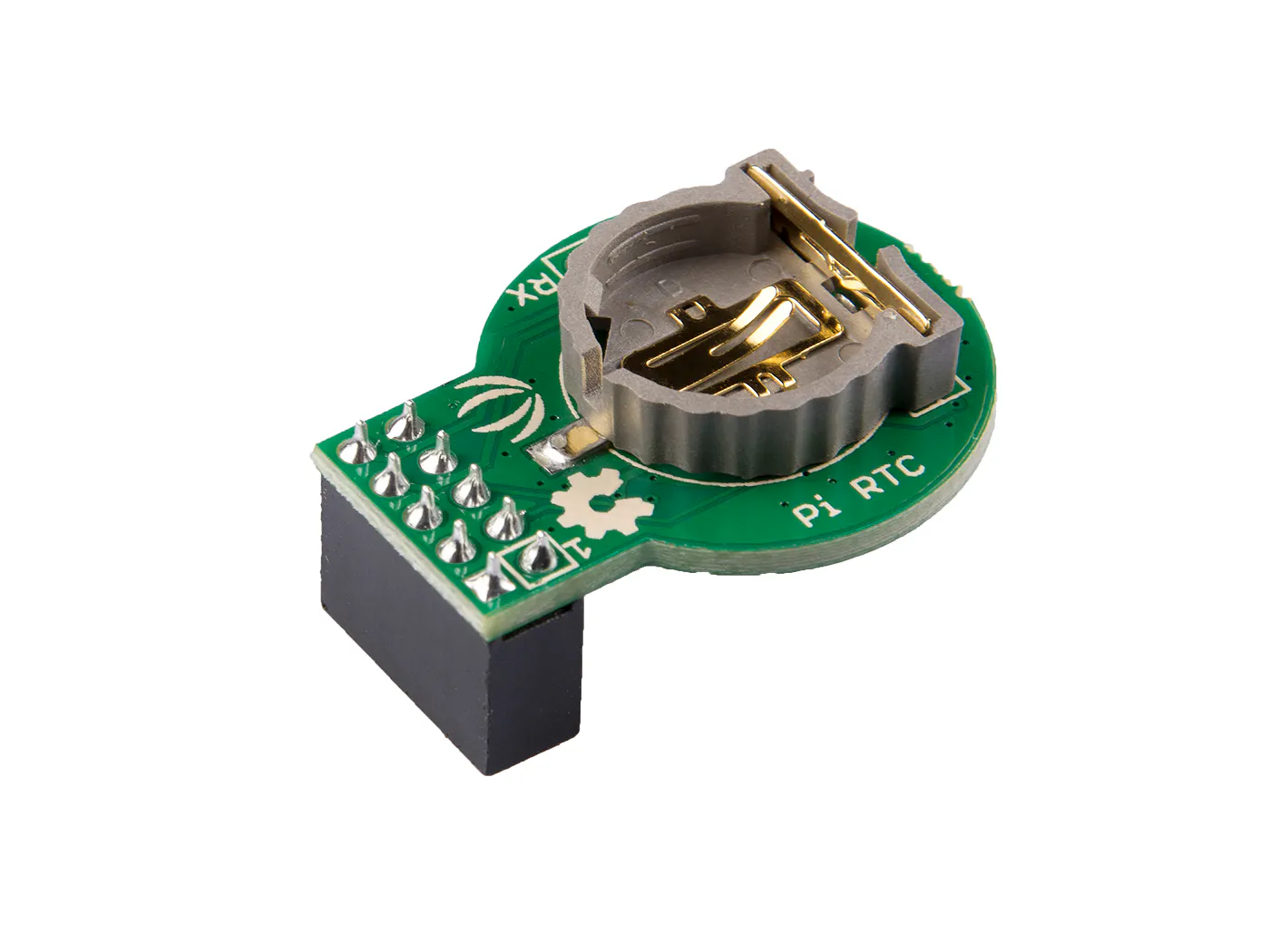Seeed Studio Pi RTC (DS1307)
×1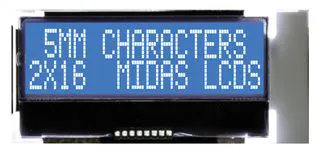Alphanumeric LCD, 16 x 2
×1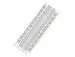×1Jumper wires (generic)
×1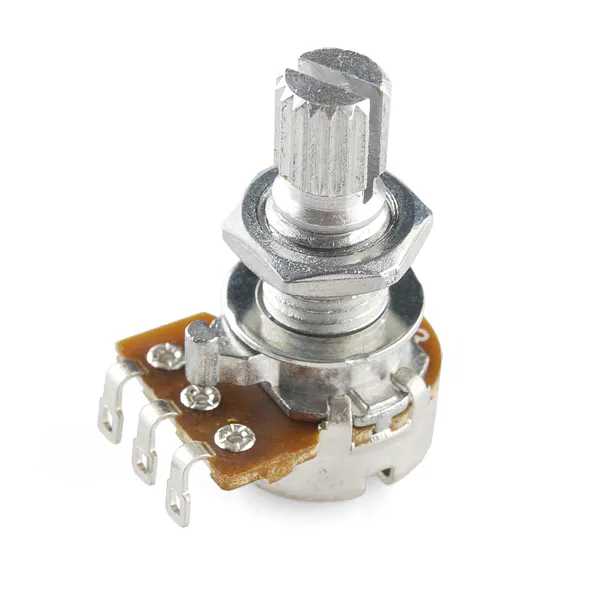Rotary potentiometer (generic)
×1Resistor 10k ohm
×2Resistor 1k ohm
×1

### Software apps and online servicesArduino IDE

## Schematics

### Arduino RTC clock schematic

connect all the hardware according to it

## Code

### Arduino DS1307 RTC code

C/C++
copy and paste on your editor
```// Real time clock and calendar with set buttons using DS1307 and Arduino

// include LCD library code
#include <LiquidCrystal.h>
// include Wire library code (needed for I2C protocol devices)
#include <Wire.h>

// LCD module connections (RS, E, D4, D5, D6, D7)
LiquidCrystal lcd(5,6,7,8,9,10);

void setup() {
pinMode(3, INPUT_PULLUP);                      // button1 is connected to pin 8
pinMode(4, INPUT_PULLUP);                      // button2 is connected to pin 9
// set up the LCD's number of columns and rows
lcd.begin(16, 2);
Wire.begin();                                  // Join i2c bus
}

char Time[]     = "TIME:  :  :  ";
char Calendar[] = "DATE:  /  /20  ";
byte i, second, minute, hour, date, month, year;

void DS1307_display(){
// Convert BCD to decimal
second = (second >> 4) * 10 + (second & 0x0F);
minute = (minute >> 4) * 10 + (minute & 0x0F);
hour   = (hour >> 4)   * 10 + (hour & 0x0F);
date   = (date >> 4)   * 10 + (date & 0x0F);
month  = (month >> 4)  * 10 + (month & 0x0F);
year   = (year >> 4)   * 10 + (year & 0x0F);
// End conversion
Time     = second % 10 + 48;
Time     = second / 10 + 48;
Time      = minute % 10 + 48;
Time      = minute / 10 + 48;
Time      = hour   % 10 + 48;
Time      = hour   / 10 + 48;
Calendar = year   % 10 + 48;
Calendar = year   / 10 + 48;
Calendar  = month  % 10 + 48;
Calendar  = month  / 10 + 48;
Calendar  = date   % 10 + 48;
Calendar  = date   / 10 + 48;
lcd.setCursor(0, 0);
lcd.print(Time);                               // Display time
lcd.setCursor(0, 1);
lcd.print(Calendar);                           // Display calendar
}
byte j = 0;
j++;
delay(25);
}
}
byte edit(byte x, byte y, byte parameter){
char text;
while(!digitalRead(3));                        // Wait until button (pin #8) released
while(true){
while(!digitalRead(4)){                      // If button (pin #9) is pressed
parameter++;
if(i == 0 && parameter > 23)               // If hours > 23 ==> hours = 0
parameter = 0;
if(i == 1 && parameter > 59)               // If minutes > 59 ==> minutes = 0
parameter = 0;
if(i == 2 && parameter > 31)               // If date > 31 ==> date = 1
parameter = 1;
if(i == 3 && parameter > 12)               // If month > 12 ==> month = 1
parameter = 1;
if(i == 4 && parameter > 99)               // If year > 99 ==> year = 0
parameter = 0;
sprintf(text,"%02u", parameter);
lcd.setCursor(x, y);
lcd.print(text);
delay(200);                                // Wait 200ms
}
lcd.setCursor(x, y);
lcd.print("  ");                             // Display two spaces
sprintf(text,"%02u", parameter);
lcd.setCursor(x, y);
lcd.print(text);
if(!digitalRead(3)){                         // If button (pin #8) is pressed
i++;                                       // Increament 'i' for the next parameter
return parameter;                          // Return parameter value and exit
}
}
}

void loop() {
if(!digitalRead(3)){                           // If button (pin #8) is pressed
i = 0;
hour   = edit(5, 0, hour);
minute = edit(8, 0, minute);
date   = edit(5, 1, date);
month  = edit(8, 1, month);
year   = edit(13, 1, year);
// Convert decimal to BCD
minute = ((minute / 10) << 4) + (minute % 10);
hour = ((hour / 10) << 4) + (hour % 10);
date = ((date / 10) << 4) + (date % 10);
month = ((month / 10) << 4) + (month % 10);
year = ((year / 10) << 4) + (year % 10);
// End conversion
// Write data to DS1307 RTC
Wire.beginTransmission(0x68);               // Start I2C protocol with DS1307 address
Wire.write(0);                              // Reset sesonds and start oscillator
Wire.write(minute);                         // Write minute
Wire.write(hour);                           // Write hour
Wire.write(1);                              // Write day (not used)
Wire.write(date);                           // Write date
Wire.write(month);                          // Write month
Wire.write(year);                           // Write year
Wire.endTransmission();                     // Stop transmission and release the I2C bus
delay(200);                                 // Wait 200ms
}
Wire.beginTransmission(0x68);                 // Start I2C protocol with DS1307 address
Wire.endTransmission(false);                  // I2C restart
Wire.requestFrom(0x68, 7);                    // Request 7 bytes from DS1307 and release I2C bus at end of reading